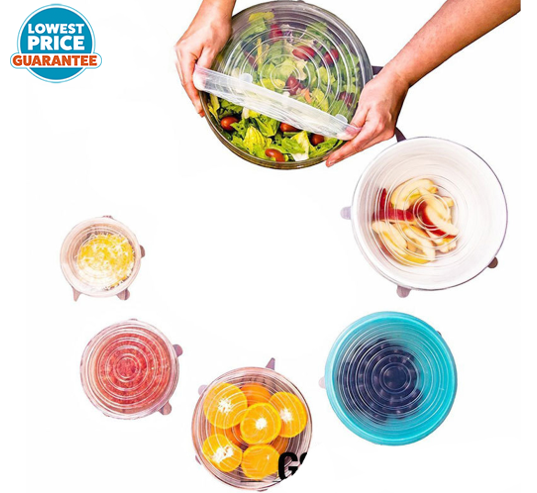ã€ D i s c o u n t P r o d u c t ã€‘ 6 P c s R e u s a b l e S a r a n W r a p S i l i c o n e C o v e r V a c u u m S e a l F o o d B o w l S e a l e r K i t c h e n P U 0 3 7

Updated Wednesday 09-Oct-2019ã€ D i s c o u n t P r o d u c t ã€‘ 6 P c s R e u s a b l e S a r a n W r a p S i l i c o n e C o v e r V a c u u m S e a l F o o d B o w l S e a l e r K i t c h e n P U 0 3 7 2

THB 97.62

More details at ezbuy.co.th